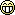The online racing simulator
Drag strip 804 meters / 1/2 mile
(4 posts, started )
Drag strip 804 meters / 1/2 mile
How fast can you get your car on this drag strip?

Start = 0
Split 1 = 201 meters / 1/8 mile
Split 2 = 402 meters / 1/4 mile
Split 3 = 603 meters / 3/8 mile
Finish = 804 meters / 1/2 mile

This measurements is 100% perfect.

Post your best time down below (WITHOUT TWEEKS)
Any car will do, or all of them.

Enjoy
Attached images
XRT: Split 1 = 8.43
Split 2 = 13.11
Split 3 = 17.16
Finish = 20.83

FXO: Split 1 = 8.92
Split 2 = 13.62
Split 3 = 17.67
Finish = 21.34

RB4: Split 1 = 8.31
Split 2 = 13.05
Split 3 = 17.13
Finish = 20.84
------------------------------------
FZ5: Split 1 = 7.71
Split 2 = 11.96
Split 3 = 15.62
Finish = 18.92

RAC: Split 1 = 7.77
Split 2 = 11.98
Split 3 = 15.64
Finish = 19.00

LX6: Split 1 = 7.67
Split 2 = 11.85
Split 3 = 15.54
Finish = 18.97
------------------------------------
XFG: Split 1 = 10.19
Split 2 = 15.80
Split 3 = 20.67
Finish = 25.10

XRG: Split 1 = 9.69
Split 2 = 15.20
Split 3 = 19.97
Finish = 24.29
------------------------------------
UF1: Split 1 = 10.97
Split 2 = 17.26
Split 3 = 22.77
Finish = 27.86

LX4: Split 1 = 8.07
Split 2 = 12.61
Split 3 = 16.65
Finish = 20.39
------------------------------------
UFR: Split 1 = 7.98
Split 2 = 12.30
Split 3 = 16.09
Finish = 19.57

XFR: Split 1 = 8.28
Split 2 = 12.66
Split 3 = 16.47
Finish = 19.95
Attached files
FZR: Split 1 = 6.61
Split 2 = 10.32
Split 3 = 13.56
Finish = 16.51

XRR: Split 1 = 6.81
Split 2 = 10.53
Split 3 = 13.78
Finish = 16.75

FXR: Split 1 = 6.58
Split 2 = 10.35
Split 3 = 13.64
Finish = 16.66
------------------------------------
MRT: Split 1 = 8.16
Split 2 = 13.12
Split 3 = 17.64
Finish = 21.92
------------------------------------
FBM: Split 1 = 7.69
Split 2 = 12.09
Split 3 = 16.00
Finish = 19.63
------------------------------------
FOX: Split 1 = 7.38
Split 2 = 11.51
Split 3 = 15.19
Finish = 18.59
------------------------------------
FO8: Split 1 = 6.38
Split 2 = 9.74
Split 3 = 12.69
Finish = 15.42
------------------------------------
BF1: Split 1 = 5.88
Split 2 = 8.71
Split 3 = 11.21
Finish = 13.50

Anyone else?
Attached files
Looks like BF1 is the way to go, me and my friend will try to make it below 13.50Drag strip 804 meters / 1/2 mile
(4 posts, started )

© 2002-2020 Live for Speed - Scawen Roberts, Eric Bailey, Victor van Vlaardingen
All timestamps on this website are displayed in your local timezone
FGED GREDG RDFGDR GSFDG